### Home > MC2 > Chapter 7 > Lesson 7.1.4 > Problem7-38

7-38.

Lydia drew a graph of four athletes in the final part of the triathlon, the 10 km run. She found the slope of each runner's line. Her results are listed below. List the runners from slowest to fastest. Homework Help ✎ Runner A: slope =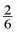Runner B: slope =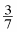Runner C: slope =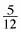Runner D: slope =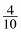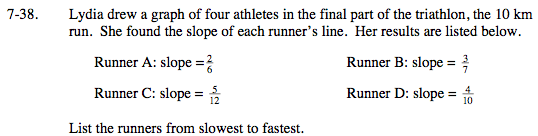The fastest athlete will have the greatest slope, because that means that they were able to run a greater distance in a given amount of time.

One way to see which runner had the greatest slope is to convert each fraction into a decimal.

The decimal approximation for Runner A is 0.33 and for Runner B it is 0.43.
Find the decimal values for the other two runners, then arrange them from least to greatest.